150804 Epaper

 A Section B SectionC Section D Section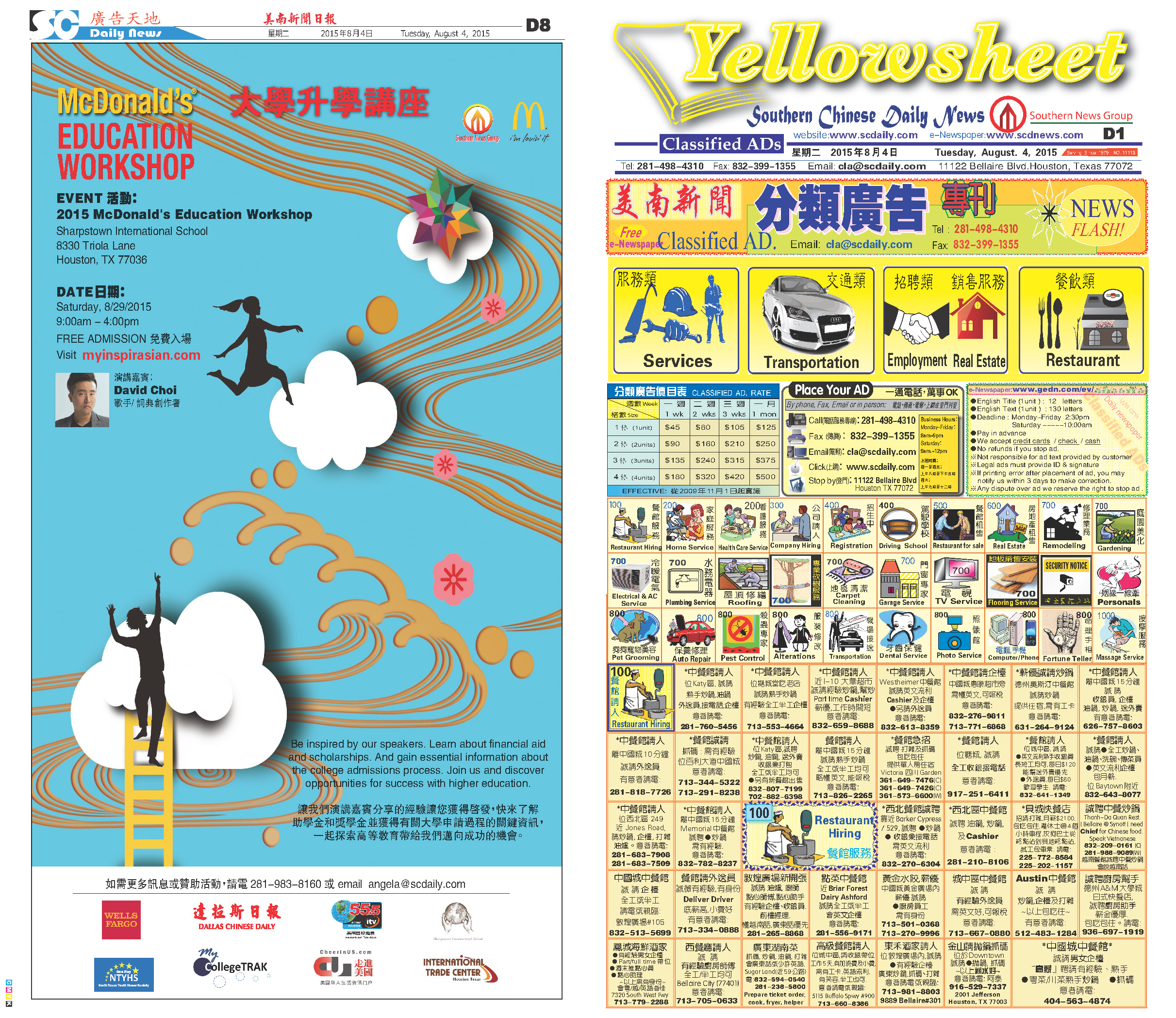150803 Epaper

 A Section B SectionC Section D Section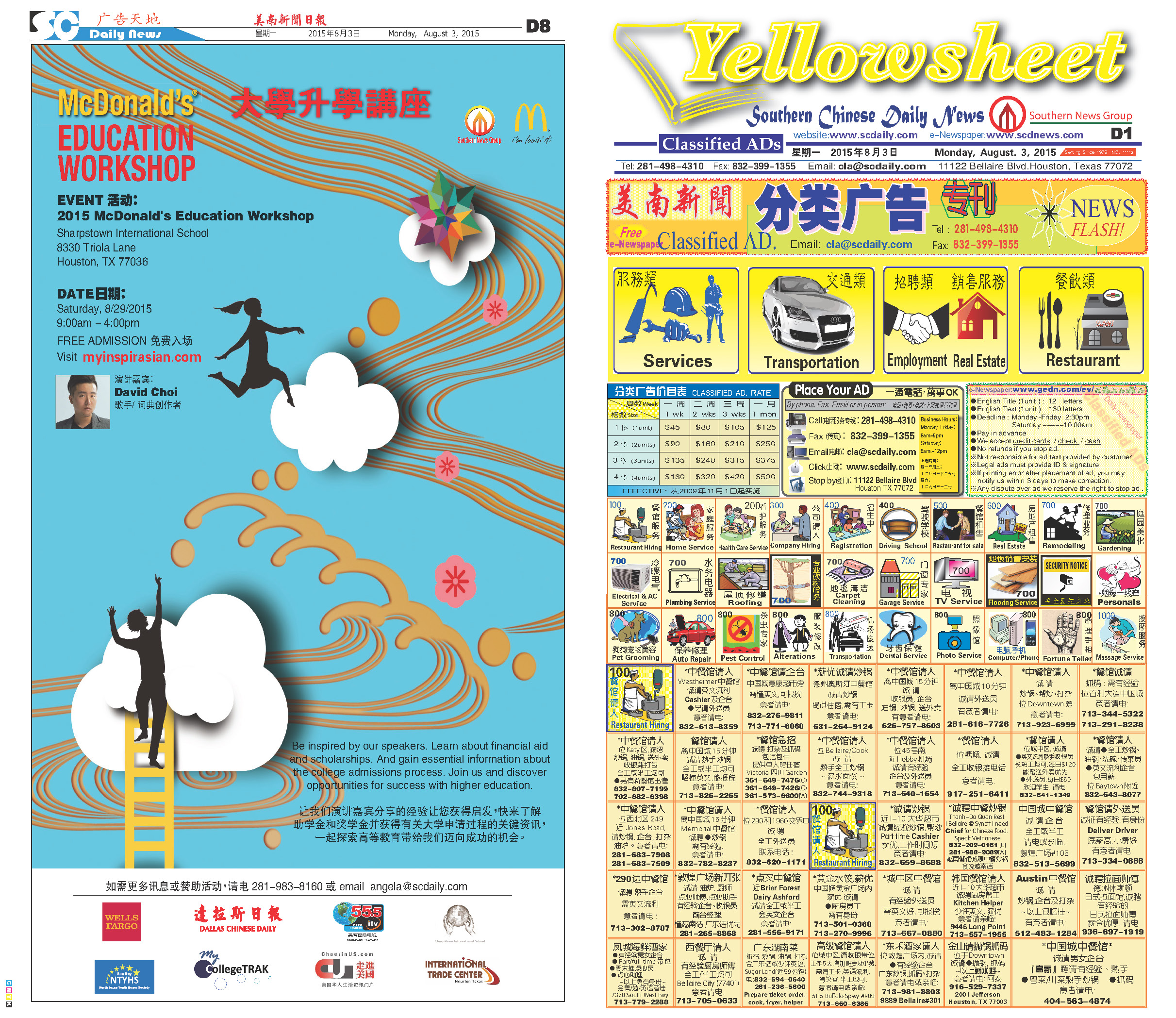150802 Epaper

 A Section B SectionC Section D Section150801 Epaper

 A Section B SectionC Section D Section150731 Epaper

 A Section B SectionC Section D Section150730 Epaper

 A Section B SectionC Section D Section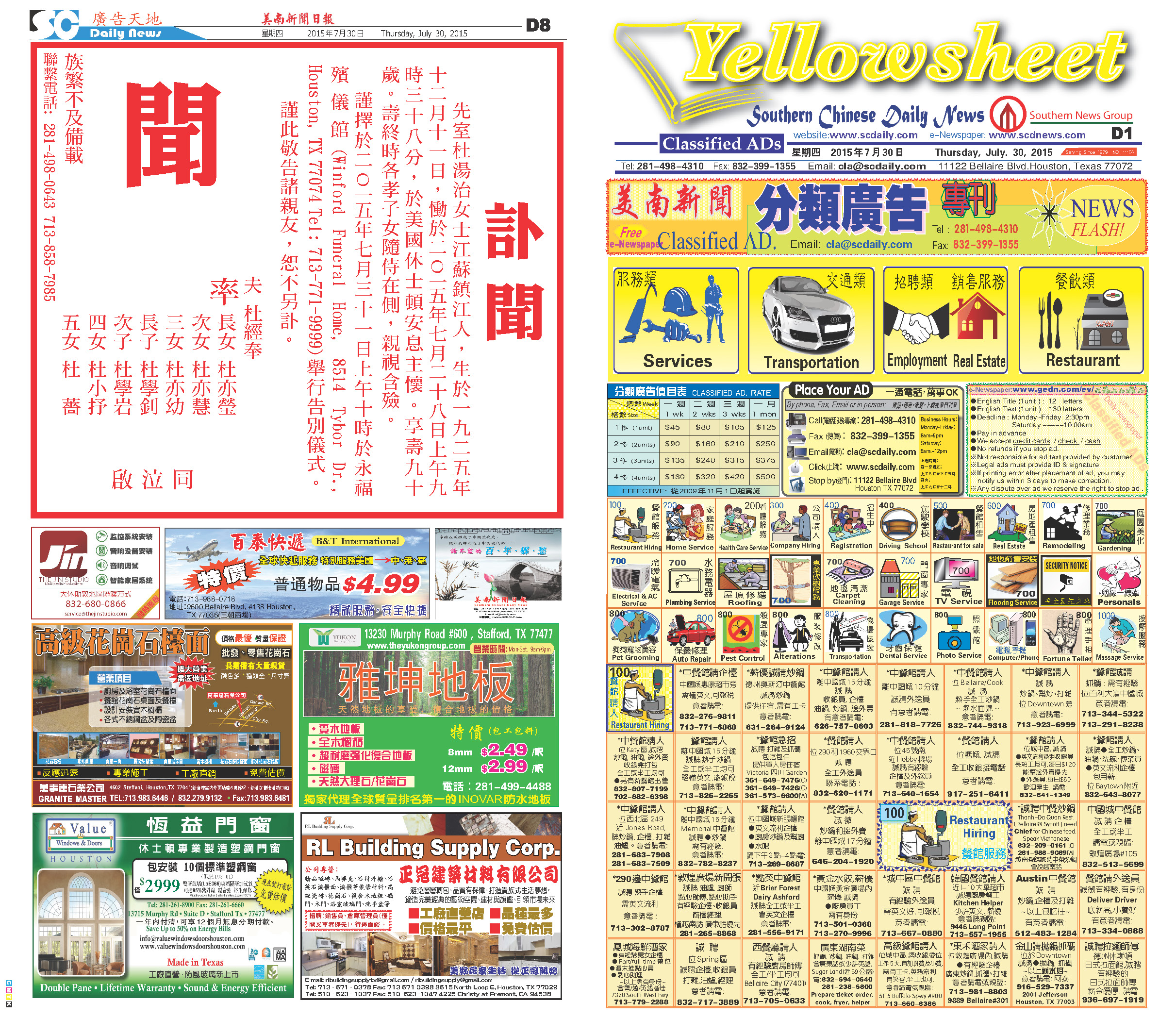150729 Epaper

 A Section B SectionC Section D Section150728 Epaper

 A Section B SectionC Section D Section150726 Epaper

 A Section B Section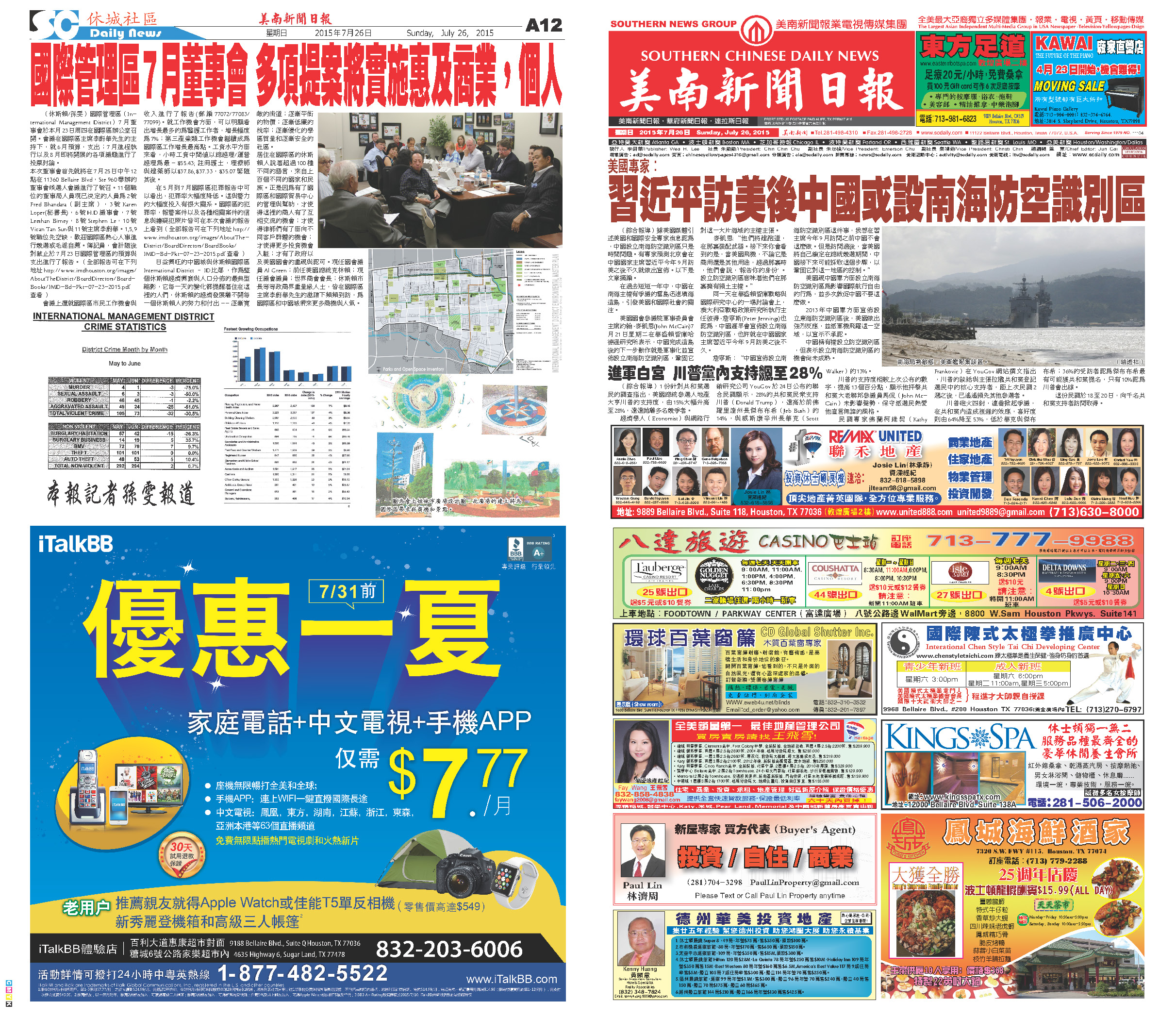C Section D Section150727 Epaper

 A Section B SectionC Section D Section150725 Epaper

 A Section B Section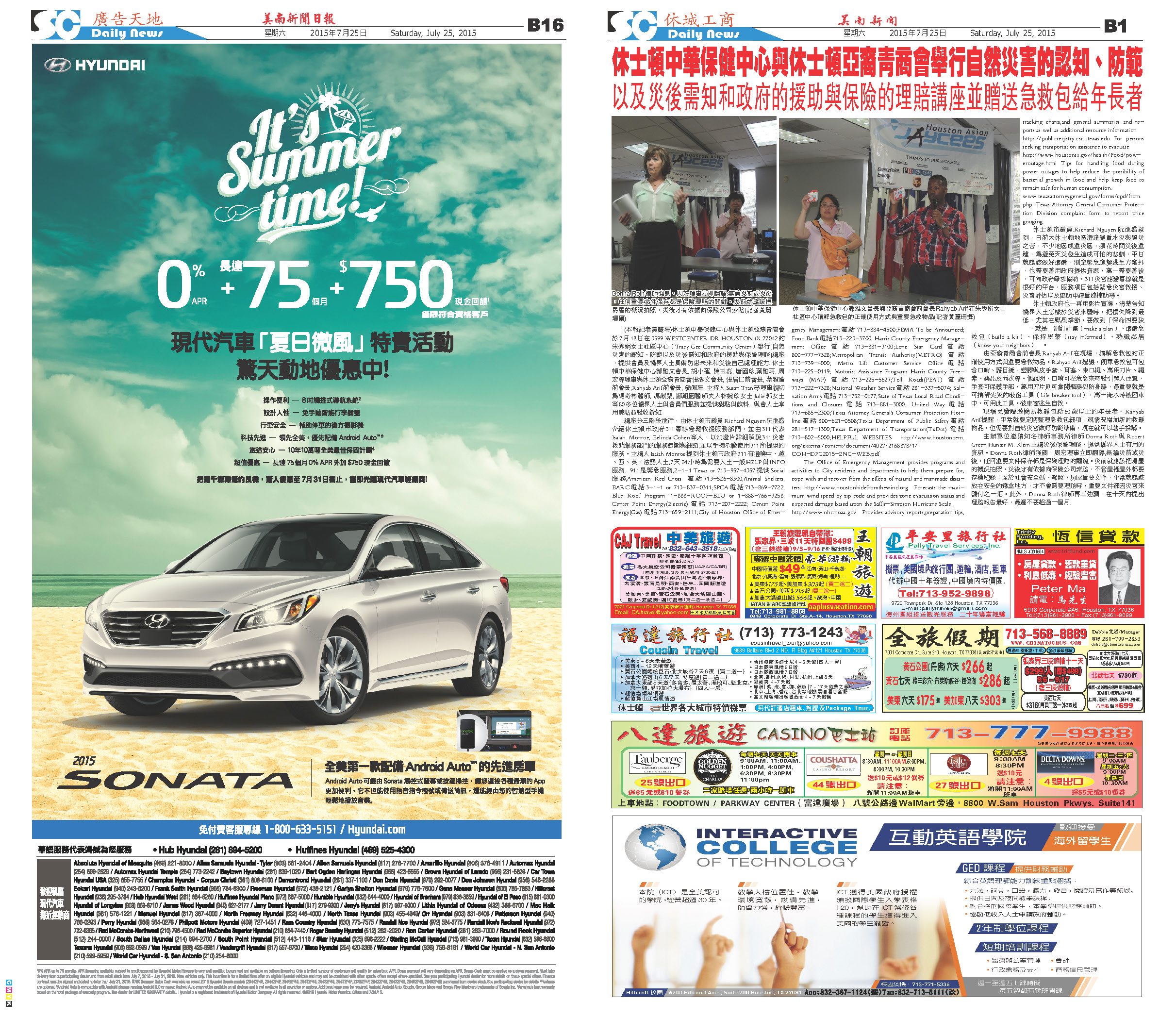C Section D Section